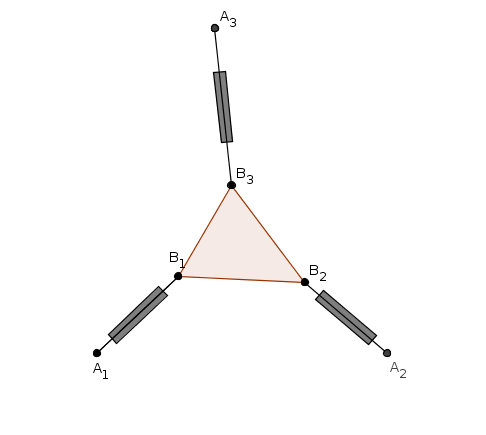## 3-RPR : the forward kinematic problem

A 3-RPR planar manipulator consists of a platform moving in a plane and linked to the base via three legs AiBi , each one consisting of:

• a passive revolute joint (R) centered at Ai ,
• an actuated prismatic joint (P) controlling the length of the leg AiBi ,
• a passive revolute joint (R) centered at Bi .If the position of the platform B1B2B3 is known, then the lengths li of the legs AiBi are known. The forward kinematic problem is the following : how many positions of the platform correspond to given (l1, l2, l3) ?

If the lengths of the legs A1B1 and A2B2 are fixed to l1 and l2 and the leg A3B3 is cut, see what happens:

The third vertex of the moving platform describes a curve which is a 3-cyclic sextic (3-cyclic means that the cyclic points belong to the curve with multiplicity 3). On the animation, the leg A1B1 rotates. To each position of this leg correspond at most  two possible positions of the leg A2B2 : one with "knee B2 up" (this mode is indicated in green) and the other with "knee B'2 down" (in red). The corresponding points B3 and B'3 are actually on the same sextic, which has two connected components in the default setting of the applet. But you can move the point A2 to see how the shape of the curve evolves and obtain eventually a situation where the sextic has only one connected component (with two segments of different colors).

The number of intersections of the sextic with the circle of center A3 and radius l3 is the number of solutions to the forward kinematic problem. You can move the point A3, change the radius l3 and check that the number of intersections is generically 0, 2, 4 or 6.

Reference : J-P. Merlet, Parallel Robots, Springer

Michel Coste, created avec GeoGebra